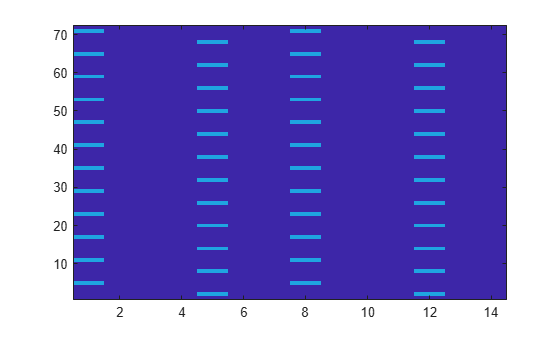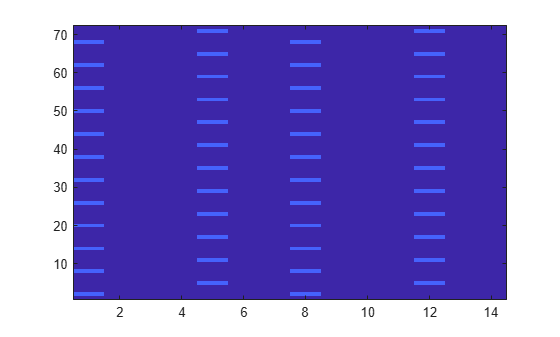# Map Reference Signal to Resource Grid

This example shows how to map the cell specific reference signals to the resource grid for a subframe in the two antenna case.

Specify the parameter structure. In this scenario, there are 6 resource blocks in the downlink.

```enb.CyclicPrefix = 'Normal'; enb.NDLRB = 6; enb.CellRefP = 2; enb.DuplexMode = 'FDD';```

Create an empty resource grid for a subframe.

`resourceGrid = lteDLResourceGrid(enb);`

Specify `NCellID` and `NSubframe` in the input parameter structure. These fields are required to generate the cell-specific reference signals. In this example, select subframe number 0.

```enb.NCellID = 10; enb.NSubframe = 0;```

Generate the cell-specific reference signals for the two antenna ports by calling the `lteCellRS` function.

```rsAnt0 = lteCellRS(enb,0); rsAnt1 = lteCellRS(enb,1);```

Generate mapping indices for the two antenna ports. You need these mapping indices to map the generated complex symbols to the resource grid.

```indAnt0 = lteCellRSIndices(enb,0); indAnt1 = lteCellRSIndices(enb,1);```

Map the reference signal complex symbols to the resource grid. Use the generated indices in linear form. Plot the resource grid.

```resourceGrid(indAnt0) = rsAnt0; resourceGrid(indAnt1) = rsAnt1; size(resourceGrid)```
```ans = 1×3 72 14 2 ```
```image(100*abs(resourceGrid(:,:,1))) axis xy``````figure image(50*abs(resourceGrid(:,:,2))) axis xy```The resulting matrix has the complex symbols in `rsAnt0` and `rsAnt1` in the appropriate locations, specified by `indAnt0` and `indAnt1`.